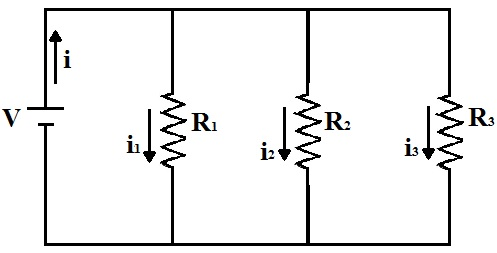Electricity & Megnetism

# Connecting resistors in parallel

In the association of resistors in parallel, the equivalent resistance is equal to the sum of the reciprocals of the resistances of the individual resistors that form the electrical circuit.

Resistors are devices that transform electrical energy into thermal energy through the E made Joule , thus dissipating the energy produced by a voltage source. When placed in electrical circuits , they are intended to limit the current flowing through the circuit. However, we can’t always find a resistor with the resistance we need, but we can make a combination of resistors to get a value equivalent to what we need. This combination is called resistor association.

Resistor association can be done in series (see Properties of series resistor association ) and in parallel. When it is done in parallel, it can be represented as follows:Representation of the association of resistors in parallel

Note that resistors R 1 , R 2 and R 3 are powered by the same voltage source V.

V = V 1 = V 2 = V 3

This makes them subject to the same potential difference (ddp), but they are traversed by different electrical currents, which are proportional to the value of each one.

Let us then consider that the electric current that passes through the resistors has the respective intensities: i 1 , i 2 and i 3 . Thus, the intensity i of the electric current supplied by the source is given by:

i = i 1 + i 2 + i 3

The ddp across each resistor is the same and can be obtained through Ohm’s law :

V = R 1 ? i 1 → i 1 = V
R 1

V = R 2 ? i 2 → i 2 = V
R 2

V = R 3 ? i 3 → i 3 = V
R 3

With the association of resistors, we obtain an equivalent resistance R eq that depends on the electric current and the voltage supplied by the source. This resistance is also obtained by Ohm’s law:

V = R eq ? i → i = V
R eq

Until now, the electric current of each of the resistors was obtained as a function of the electric current and the voltage supplied by the source. Substituting these values ​​into the previous equation, we can find the relationship between the three resistors:

i = i 1 + i 2 + i 3

V = V + V + V
eq   R 1    R 2   R 3

Simplifying V, we have:

1 = _ 1_ + _ 1_ + _ 1_
eq     R 1      R 2       R 3

This expression is valid for any number of resistors associated in parallel. Therefore, it can be stated as follows:

Don’t stop now… There’s more after the publicity 😉

“ The equivalent resistance R eq of a circuit containing resistors R 1 , R 2 , R 3 , …, R n, , connected in parallel to a voltage source, is given by the formula:

1 = _ 1_ + _ 1_ + _ 1_ + … + _ 1_
R eq   R 1         R 2        R 3                 n

that is, the inverse of the equivalent resistance of the circuit is equal to the sum of the inverses of the resistances of resistors connected in parallel.”

Some special cases of connecting resistors in parallel

Depending on the number of resistors and the value of their resistances, we can simplify the expression used to calculate the equivalent resistance:

• Equivalent resistance of two resistors in parallel

In this case, the equivalent resistance is given by:

1 = _ 1_ + _ 1_
eq   R 1       R 2

The MMC between R 1 and R 2 is given by the product between them, remembering that, when dividing a fraction, we must divide the MMC by the denominator and multiply by its respective numerator. Thus, we obtain the expression:

1 = 1 + R 2
eq R 1 ? R 2

Cross-multiplying to isolate R eq , we arrive at the equation:

eq = 1 ? R 2
1 + R 2

This formula is a simplification of the calculations and, through it, we can directly substitute the resistance values.

• Resistors with equal values

Suppose there is an association with three resistors of values ​​equal to R in parallel. The equivalent resistance is given by the following expression:

_1 = _ 1_ + _ 1_ + _ 1_
R eq      R R R

Knowing that, in adding fractions with like denominators, we conserve the denominators and add the numerators, the above equation can be rewritten as:

_1 = _ 3_
R eq    R

Isolating the equivalent resistance, we get the equation:

eq = R
3

For any number of resistors connected in parallel whose individual resistors value the same, we calculate the equivalent resistance by dividing the value of one resistor by the number of resistors in the circuit.

Properties of the association of resistors in parallel

In the association of resistors in parallel, the equivalent resistance is always smaller than the smallest resistance in the circuit.

When one of the resistors in the parallel association burns out, the electric current flowing in the other components of the circuit does not change.

By virtue of this second property, residential electrical circuits and public lighting are all in parallel. If they were in series, when a light bulb in a room stopped working, all the other light bulbs would stop as well, as this would prevent the flow of electric current.